Whole Number Line Plots
Fraction Line Plots
Volume
100

Use the following line plot to answer the question: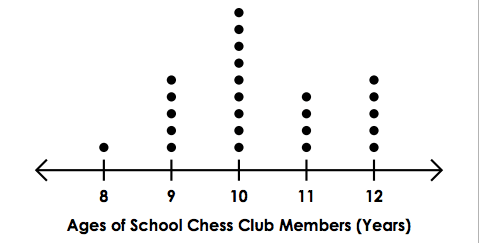What is the most common age?

10 years old

100

In this data set:

4, 4, 3, 6, 3, 2

What is the total of the 3 smallest values combined?

100

Use the following line plot to answer the question: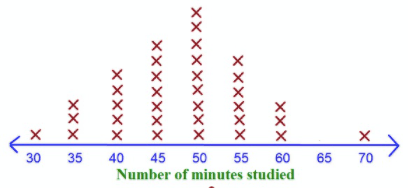What was the LEAST common number of minutes studied?

30 and 70 minutes.

100

Use the following line plot to answer the question:What is the total amount of juice in cups that is GREATER than 2?

7 cups

100

What units should volume be written as?

Cubic Units / Units Cubed

200

Use the following line plot to answer the question:What is the age of the youngest Chess Club Member?

8 years old

200

Look at the following data set:

8, 6, 5, 5, 3, 4, 3, 6, 7, 5, 6, 7, 8

On a number line, how many X's would be placed above the number 5?

3 X's

200

Use the following line plot to answer the question: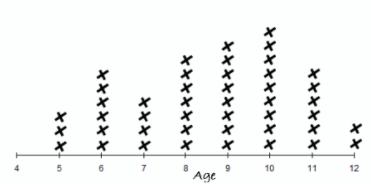What is the most common age?

10

200

Use the following line plot to answer the question: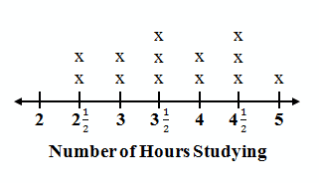John tracked the number of hours he studied for each of his study sessions leading up to his final. How many times did he have a study session?

13 study sessions

200

what are the 2 formulas to find volume?

L * W * H

Area of Base * Height

300

Use the following line plot to answer the question:What is the age of the oldest Chess Club Member?

12 years old

300

Look at the following data set:

8, 6, 5, 5, 3, 4, 3, 6, 7, 5, 6, 7, 8

Which number is the LEAST common?

4

300

Use the following line plot to answer the question:What is the most common number?

3

300

Use the following line plot to answer the question: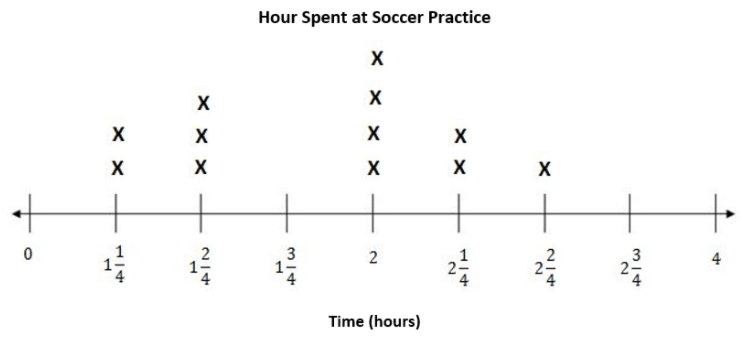What was the most common time spent at soccer practice?

2 hours

300
A box is 8 feet wide 6 feet tall. The Volume is 192 cubic feet. How long is the box?

4 feet

400

Use the following line plot to answer the question:How many Chess Club Members are younger than 11 years old?

15 Chess Club Members

400

Make a line plot using the following data set:

5, 4, 5, 6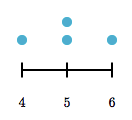400

Use the following line plot to answer the question: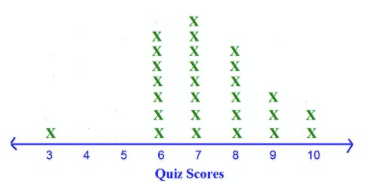How many people scored higher than 7 on the quiz?

11

400

Use the following line plot to answer the question: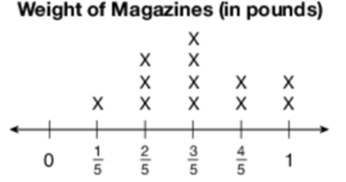What is the difference between the heaviest and the lightest magazines?

4/5 pounds

400

What is the volume of a room that has an area of 74 sq feet and a height of 7 ft?

518 cubic feet

500

Use the following line plot to answer the question:How many total members are in the Chess Club?

24 Members

500

In this data set: what is the value of the 4 largest values combined?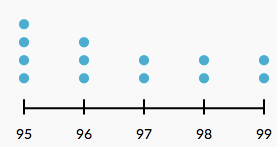394

500

Use the following line plot to answer the question: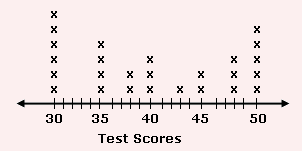What is the difference between the highest and the lowest test scores?

50 - 30 = 20

500

Use the following line plot to answer the question: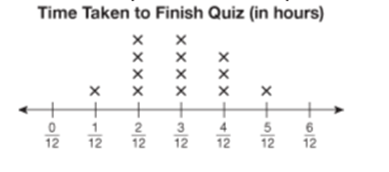What was the total amount of time it took to finish the quiz for people who took longer than 3/12 hours?

1 5/12 hours

500

What is the volume of a house that is 62 ft wide and 217 ft long and 8ft tall?

107,632 ft CUBED

Click to zoom